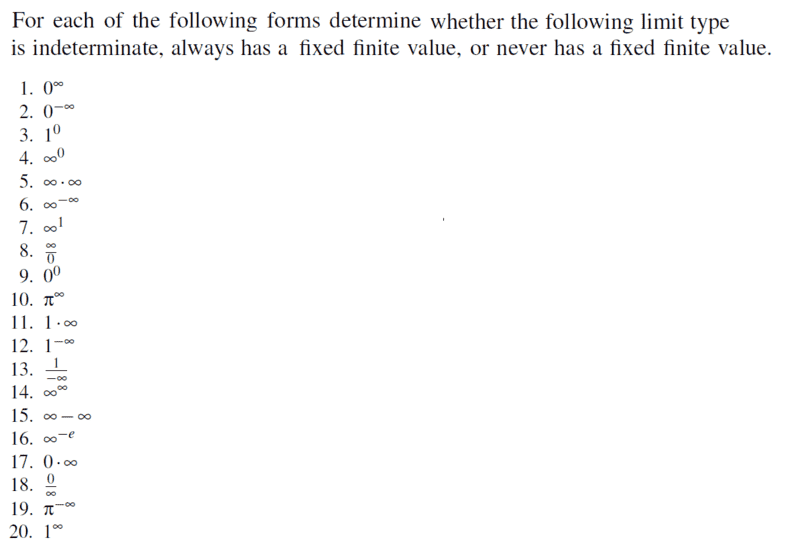# Determine whether limit is indeterminate or has a fixed value

• songoku
In summary, the following are all indeterminate forms: ##\frac{0}{0}, \frac{\infty}{\infty} , \infty - \infty, 0 . \infty , 1^{\infty}, 0^{0}, \infty^{0}##

#### songoku

Homework Statement
Relevant Equations
Indeterminate formsIndeterminate forms are: ##\frac{0}{0}, \frac{\infty}{\infty} , \infty - \infty, 0 . \infty , 1^{\infty}, 0^{0}, \infty^{0}##

4, 9, 15, 17, 20 are inderterminate forms

1. always has a fixed finite value, which is zero

2. ##0^{-\infty}=\frac{1}{0^{\infty}}=\frac{1}{0}=\infty## so it never has a fixed finite value

3. always has a fixed finite value, which is one

5. ##\infty . \infty=\infty## so it never has a fixed finite value

6. ##\infty^{-\infty}=\frac{1}{\infty^{\infty}}=\frac{1}{\infty}=0## so it always has a fixed finite value

7. ##\infty^{1}=\infty## so it always has a fixed finite value

8. ##\frac{\infty}{0}=\infty## so it never has a fixed finite value

10. ##\pi^{\infty}=\infty## so it never has a fixed finite value

11. ##1.\infty=\infty## so it never has a fixed finite value

12. ##1^{-\infty}=\frac{1}{1^{\infty}}## , not sure about this one since ##1^{\infty}## is indeterminate form. My guess for this one is: ##\frac{1}{1^{\infty}}=\frac{1}{1}=1## so always has a fixed finite value

13. ##\frac{1}{-\infty}=0## so it always has a fixed finite value

14. ##\infty^{\infty}=\infty## so it never has a fixed finite value

16. ##\infty^{-e}=\frac{1}{\infty^{e}}=\frac{1}{\infty}=0## so it always has a fixed finite value

18. ##\frac{0}{\infty}=0## so it always has a fixed finite value

19. ##\pi^{-\infty}=\frac{1}{\pi^{\infty}}=0## so it always has a fixed finite value

Thanks

#### Attachments

•songoku
PeroK said:
Would the answer be "indeterminate" because ##1^{\infty}## is indeterminate form?

Thanks

songoku said:
Would the answer be "indeterminate" because ##1^{\infty}## is indeterminate form?

Thanks
Why not?

PeroK said:
Why not?
I am not sure because in the explanation there is ##\lim_{x \rightarrow \infty} 1^{x} = 1## so I am confused whether it would be indeterminate form or 1

songoku said:
I am not sure because in the explanation there is ##\lim_{x \rightarrow \infty} 1^{x} = 1## so I am confused whether it would be indeterminate form or 1
Your confusion would be resolved if you found an example where it is not ##1##.

I fail to see how ##1^{\infty}## can be indeterminate, yet ##\dfrac 1 {1^{\infty}}## have a definite limit. That's a clear contradiction, is it not?

•songoku
songoku said:
I am not sure because in the explanation there is ##\lim_{x \rightarrow \infty} 1^{x} = 1## so I am confused whether it would be indeterminate form or 1

What about $\lim_{x \to \infty} (1 + kx^{-1})^x = e^{k}$? Is that of the form $1^{\infty}$?

•songoku and SammyS
PeroK said:
Your confusion would be resolved if you found an example where it is not ##1##.
You mean the one given in post #7?

pasmith said:
What about $\lim_{x \to \infty} (1 + kx^{-1})^x = e^{k}$? Is that of the form $1^{\infty}$?
I think I get your hint

Maybe a bit tricky, in that an indeterminate ##\frac {\infty}{\infty} ## may be resolved by, e.g., L' Hopital's rule.

•songoku
Thank you very much for the help and explanation PeroK, pasmith, WWGD